##### Linear Algebra For Dummies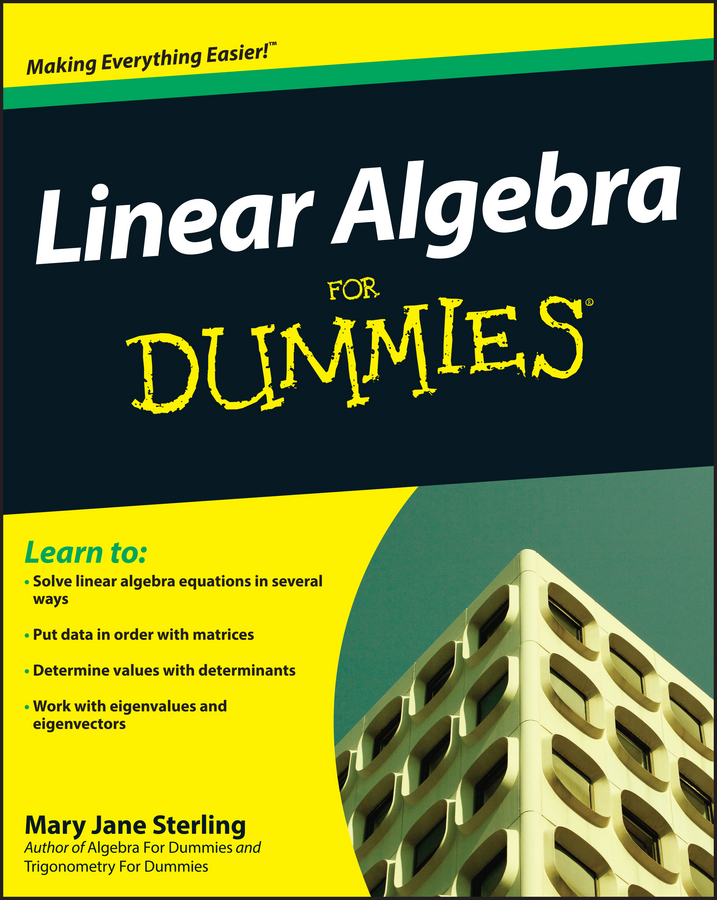The first formula most encounter to find the area of a triangle is A = 12bh. To use this formula, you need the measure of just one side of the triangle plus the altitude of the triangle (perpendicular to the base) drawn from that side. The triangle below has an area of A = 12(6)(4) = 12 square units.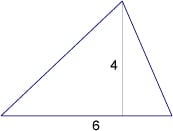Finding a perpendicular measure isn’t always convenient, especially if you’re computing the area of a large triangular piece of land, so Heron’s formula can be used to find the area of a triangle when you have the measures of the three sides. Heron’s formula uses the semi-perimeter (one-half the perimeter) and the measures of the three sides: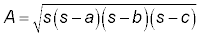where s is the semi-perimeter and a, b, and c are the measures of the sides. Finding the area of the triangle below: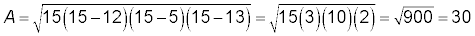(Of course, this is a right triangle, so you could just use the two perpendicular sides as base and height.)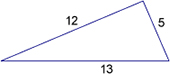Now, consider a triangle that’s graphed in the coordinate plane. You can always use the distance formula, find the lengths of the three sides, and then apply Heron’s formula. But there’s an even better choice, based on the determinant of a matrix. Here’s a formula to use, based on the counterclockwise entry of the coordinates of the vertices of the triangle (x1, y1), (x2, y2), (x3, y3) or (2, 1), (8, 9), (1, 8): A = (x1y2 + x2y3 + x3y1x1y3x2y1x3y2)/2.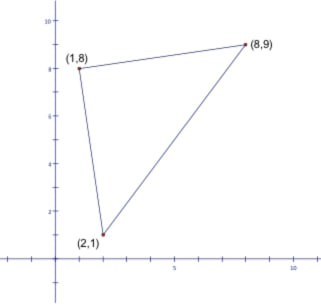Starting with the point (2, 1) and moving counterclockwise, A = (2(9) + 8(8) + 1(1) – 2(8) – 8(1) – 1(9))/2 = (18 + 64+ 1 – 16 – 8 – 9 )/2= (83 – 33)/2 = 25. The area of the triangle is 25 square units.